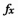# See the sum & average

You can quickly calculate the sum, average, and count in Google Sheets.

2. Highlight the cells you want to calculate.
3. At the bottom, you’ll see a row of calculations.
• Sum
• Average
• Maximum
• Minimum
• Count
4. To add a calculation to a cell, drag it to a cell.
5. Optional: To hide the row of calculations, tap Formula.